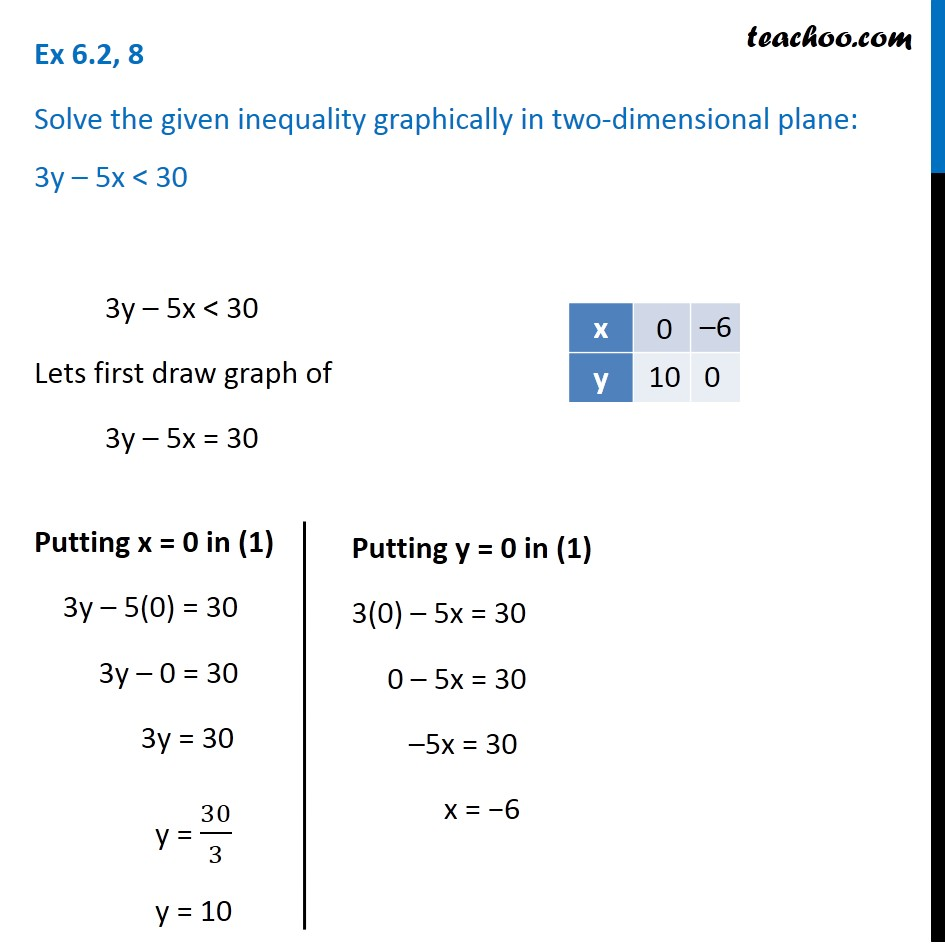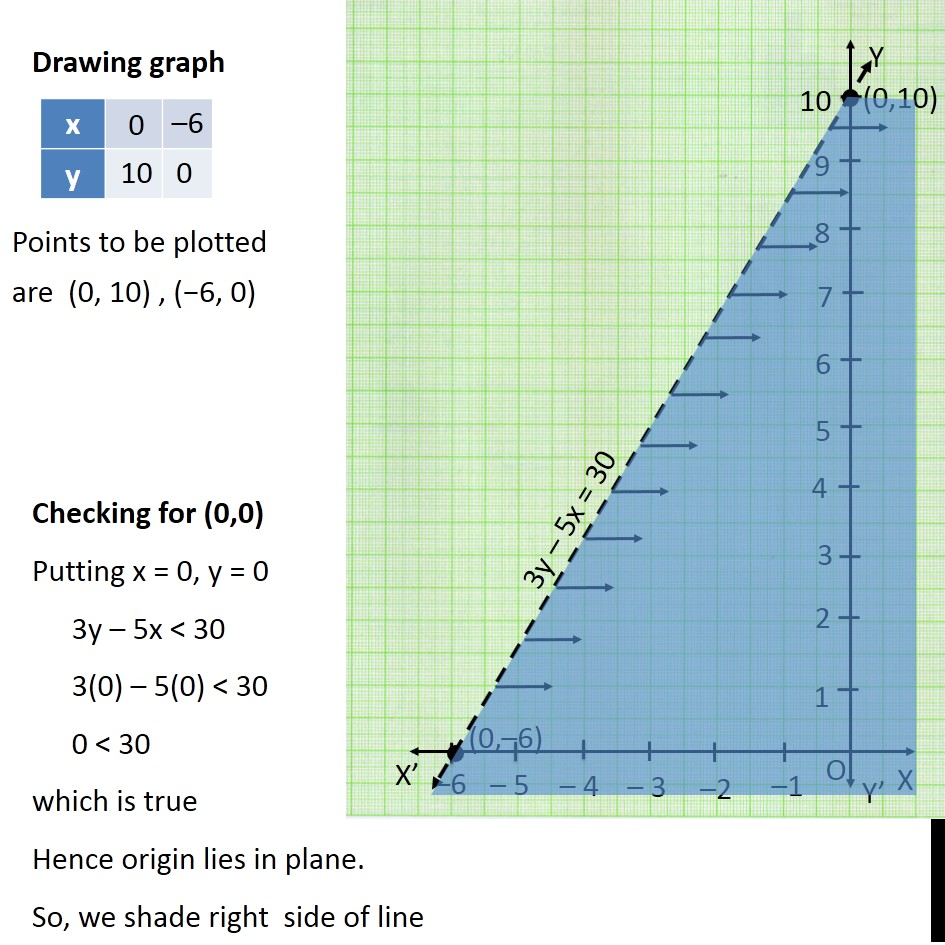Solving Linear Inequality Graphically

Chapter 5 Class 11 Linear Inequalities
Serial order wiseLearn in your speed, with individual attention - Teachoo Maths 1-on-1 Class

### Transcript

Question 8 Solve the given inequality graphically in two-dimensional plane: 3y – 5x < 30 3y – 5x < 30 Lets first draw graph of 3y – 5x = 30 Putting x = 0 in (1) 3y – 5(0) = 30 3y – 0 = 30 3y = 30 y = 30/3 y = 10 Putting y = 0 in (1) 3(0) – 5x = 30 0 – 5x = 30 –5x = 30 x = −6 Points to be plotted are (0, 10) , (−6, 0) Drawing graph Checking for (0,0) Putting x = 0, y = 0 3y – 5x < 30 3(0) – 5(0) < 30 0 < 30 which is true Hence origin lies in plane. So, we shade right side of line